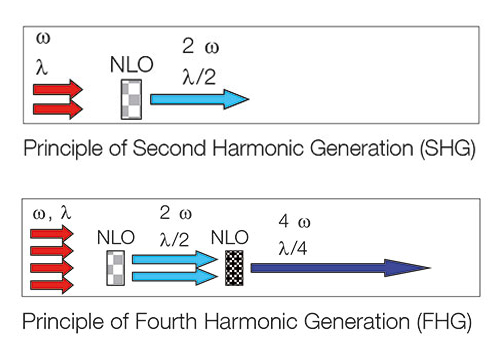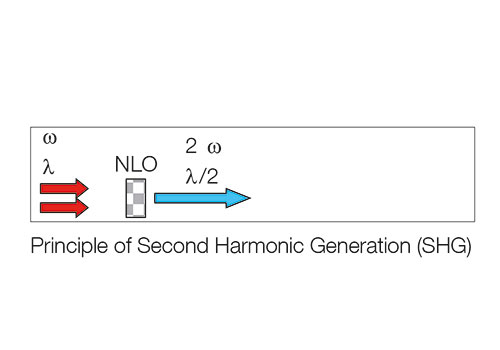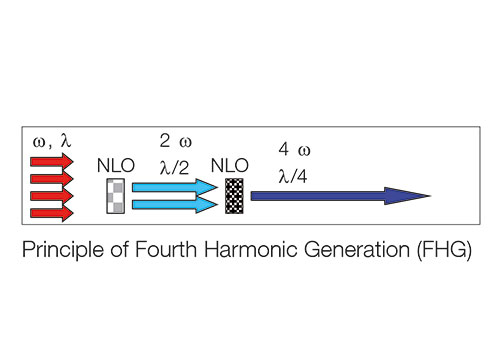## Frequency Conversion

### Closing the spectral gaps

Despite the impressive success story of semiconductor lasers some spectral “gaps”, i.e. wavelength regions, cannot be directly accessed with current laser diode technology. Nonlinear frequency conversion techniques – like Second Harmonic Generation (SHG) or Sum Frequency Generation (SFG) – close these gaps by generating laser radiation in the UV, blue, green, yellow, orange and red spectral ranges.

•••Second Harmonic Generation

TOPTICA's frequency-converted laser systems utilize second-harmonic generation (SHG). The SHG process can be explained in the wave or in the photon picture. In the wave picture, the fundamental electromagnetic wave with frequency ω1 drives the polarization of a non-linear optic (NLO) crystal. Due to the non-linearity, the polarization oscillates also at the “second harmonic” frequency ω2 = 2·ω1. This in turn leads to the emission of coherent radiation in form of of an electromagnetic wave at the frequency ω2.

In the photon picture, two photons of the fundamental laser wavelength λ1 are converted inside a NLO crystal into one photon with half the original wavelength λ2 = 0.5·λ1. The SHG efﬁciency increases with the fundamental power, the non-linearity of the utilized crystal and proper phase matching.

Phase matching

Efficient frequency conversion requires the refractive indices of the fundamental laser and the frequency-converted light to be equal within the NLO crystal. In the wave picture this leads to a constructive
interference of second-harmonic partial waves generated at different positions within the crystal along the direction of light propagation.

In the photon picture - phase matching fulfills momentum conservation: two photons of momentum p1 are converted into one photon of momentum p2 = 2·p1.

To circumvent the omnipresent dispersion which usually prevents phase matching, birefringent crystals and different polarizations of the individual lasers are used. Depending on crystal type and operating wavelength, fine-tuning of the phase matching is accomplished by proper adjustment of the crystal temperature or the angle between crystal axis and light propagation.

Resonant enhancement

TOPTICA's frequency conversion systems use a compact and rugged bow-tie resonator design with optimized mirror coatings to resonantly enhance the fundamental laser power by a factor of 50-400. The resonator length is actively stabilized with respect to the wavelength of the fundamental
laser employing the Pound-Drever-Hall method. This allows for tight locking with high long-term stability and thus reliably enhances the intra-resonator power of the fundamental laser. Together with AR-coated, temperature-controlled NLO crystals, highest conversion efficiencies in the market are achieved.

Fourth Harmonic Generation
Whilst second harmonic generation refers to frequency-doubling, fourth harmonic generation (FHG) is a process in which the frequency of an electromagnetic wave is quadrupled. Typically, the FHG process is
split into two consecutive processes, each involving a standard SHG process. In the photon picture presented before, four photons of the fundamental laser with frequency ω1 are converted into two photons with frequency ω2 = 2·ω1 in a first SHG step. A second SHG step transforms these two photons into one photon with frequency ω4 = 2·ω2 = 4·ω1 and wavelength λ4=0.25·λ1. In total, four fundamental photons disappear in the FHG process while one photon is created in compliance with energy and momentum conservation.

TOPTICA’s technical realization of such an FHG process incorporates two cascaded SHG stages, each involving actively stabilized resonant enhancement and phase matching in selected, temperature-controlled NLO crystals. Using specifically modified TA-FHG systems, TOPTICA has demonstrated cw laser radiation down to 191 nm (Scholz et al., Optics Express 20, 18659 (2012)) with more than 15 mW at 193 nm (Scholz et al., Appl. Phys. Lett. 103, 051114 (2013)).